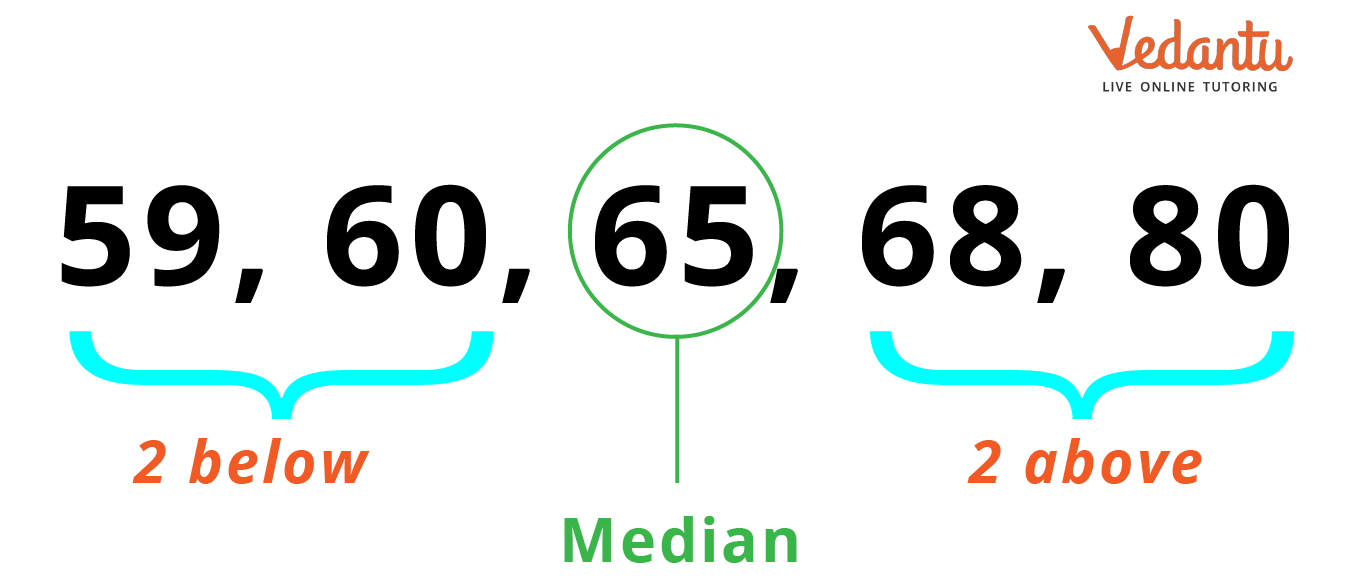Courses
Courses for Kids
Free study material
Free LIVE classes
More

# Median for an Even Number of Observations## How to Find the Median of an Even Number of Observations?

In statistics, the median of a group of observations is the value in the middle of the given data, where half of the data lies above it, and the other half lies below it. The group of observations or the data is sometimes grouped or ungrouped. The number of observations is also of two types; an odd and an even number of observations. Today we will learn about the median for even numbers of both grouped and ungrouped data.Median for a Given Data

## The Formula of the Median for Even Numbers

The median of the even number of observations is $(\dfrac{{(\dfrac{n}{2})th + (\dfrac{{n + 1}}{2})th}}{2})$, where n stands for the number of observations. If the number of observations is even, for example, 8, 12, 14, etc., then you have to use the above formula to find the median.

This is how you can calculate the value of the median for even numbers.

## Questions Related to Median for Even Numbers

As you have learnt about the median formula for even numbers of data, here are some problems that will help you understand the formula better.

1. Find the median of the given data; “24, 33, 22, 30, 21, 25, 34, and 27"

Solution:

The number of observations in the given question is 8. Hence, you must use the median formula for an even number of data points.

The next step is to arrange the given data in ascending order. Hence, the order of the numbers will be 21, 22, 24, 25, 27, 30, 33, and 34.

The value of n is 8.

Following the formula for the median for an even number of observations, you can follow the steps given below.

$\begin{array}{l}\dfrac{{{{(\dfrac{n}{2})}^{th}}observation + {{(\dfrac{{n + 1}}{2})}^{th}}observation}}{2}\\ = \dfrac{{{{(\dfrac{8}{2})}^{th}}observation + {{(\frac{{8 + 1}}{2})}^{th}}observation}}{2}\\ = \dfrac{{{4^{th}}observation + {{(4 + 1)}^{th}}observation}}{2}\end{array}$

Since the values of the 4th observation and the (4+1)th observation, that is, the 5th observation, are 25 and 27, respectively, we will replace these values in the above equation.

$\begin{array}{l} = \dfrac{{25 + 27}}{2}\\ = \dfrac{{52}}{2}\\ = 26\end{array}$

Hence, the median of the given data is 26.

2. Find the median of the given data; “2, 3, 4, 5, 6, and 7”

Solution:

The number of observations in the given question is 6, which is an even number.

We will first arrange the given data in ascending order.

As the data is already grouped, we can proceed.

We will use the formula of the median for even numbers.

The value of n=6

$\begin{array}{l}\dfrac{{{{(\dfrac{n}{2})}^{th}}observation + {{(\dfrac{{n + 1}}{2})}^{th}}observation}}{2}\\ = \dfrac{{{{(\dfrac{6}{2})}^{th}}observation + {{(\dfrac{{6 + 1}}{2})}^{th}}observation}}{2}\\ = \dfrac{{{3^{rd}}observation + {{(3 + 1)}^{th}}observation}}{2}\end{array}$

Since the values of the 3rd observation and (3+1)th observation, i.e., the 4th observation, are 4 and 5, respectively, you have to replace these values in the above equation.

$\begin{array}{l} = \dfrac{{4 + 5}}{2}\\ = \dfrac{9}{2}\\ = 4.5\end{array}$

3. Find the median of the given data; “100, 80, 90, and 40”

Solution:

The number of observations in the given question is 4, which is even.

Hence, we will arrange the given data in ascending order. Hence, the order of the given data will be 40, 80, 90, and 100.

We will use the median for even numbers formula for the question.

Since n = 4, we will replace its value in the formula.

$\begin{array}{l}\dfrac{{{{(\dfrac{n}{2})}^{th}}observation + {{(\dfrac{{n + 1}}{2})}^{th}}observation}}{2}\\ = \dfrac{{{{(\frac{4}{2})}^{th}}observation + {{(\dfrac{{4 + 1}}{2})}^{th}}observation}}{2}\\ = \dfrac{{{2^{nd}}observation + {{(2 + 1)}^{rd}}observation}}{2}\end{array}$

Since the values of the 2nd and 3rd observations are 80 and 90, respectively, we will replace these values in the above equation.

$\begin{array}{l} = \dfrac{{80 + 90}}{2}\\ = \dfrac{{170}}{2}\\ = 85\end{array}$

### Conclusion

So, today you have learnt about the median, its definition, and the two types of observation, which are odd number of observations and even number of observations. You have also learnt the formula to find out the value of the median for an even number of observations. By following these few steps, you can easily get the value of the median.

Last updated date: 26th Sep 2023
Total views: 78.3k
Views today: 1.78k

## FAQs on Median for an Even Number of Observations

1. Is it necessary for the value of the median to always be a whole number?

No, it is unnecessary for the median value to always be a whole number. As you have seen in the second question, the value was 4.5, which is not a whole number but a decimal. Therefore, the value of the median can be anything for both odd and even numbers of observations.

2. Can we find the median value of given observations without a formula?

We sometimes need the formula to find the median of an observation. Sometimes, if the number of observations is small, we can just add the two middle values of the observations and divide their sum by 2. However, following the standard process will help you avoid making mistakes.

3. Is the median formula for both odd and even observation numbers the same?

The formulas for the median of an odd number of observations and the median of an even number of observations are different. Apart from the differences in the formulas, one can get the value of an odd number of observations just by arranging the data and getting the median, which is located in the middle. However, we cannot do the same for an even number of observations.